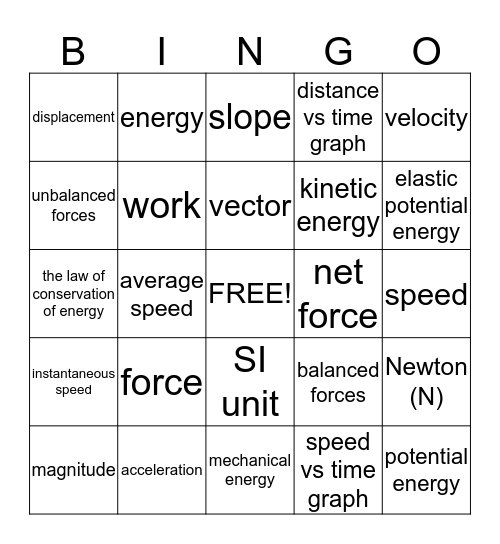# Velocity, Acceleration and FORCEThis bingo card has a free space and 24 words: force, acceleration, vector, magnitude, velocity, displacement, unbalanced forces, energy, net force, SI unit, kinetic energy, the law of conservation of energy, balanced forces, Newton (N), work, speed vs time graph, speed, slope, elastic potential energy, average speed, potential energy, instantaneous speed, distance vs time graph and mechanical energy.

## Play Online